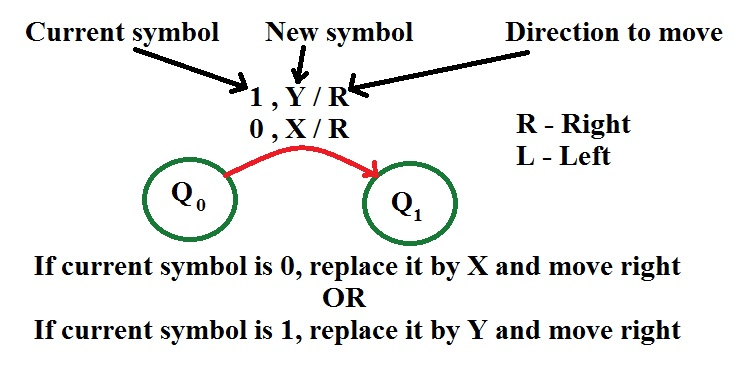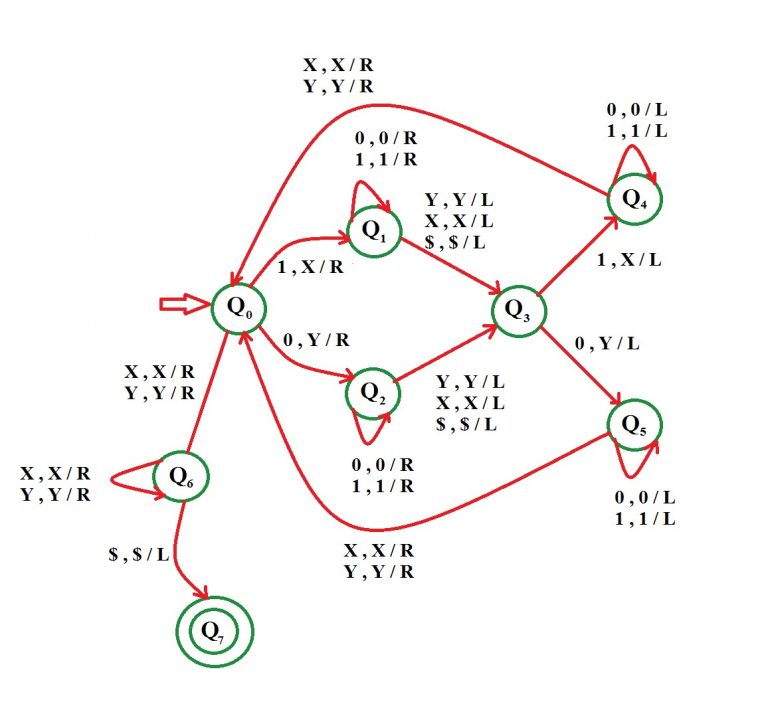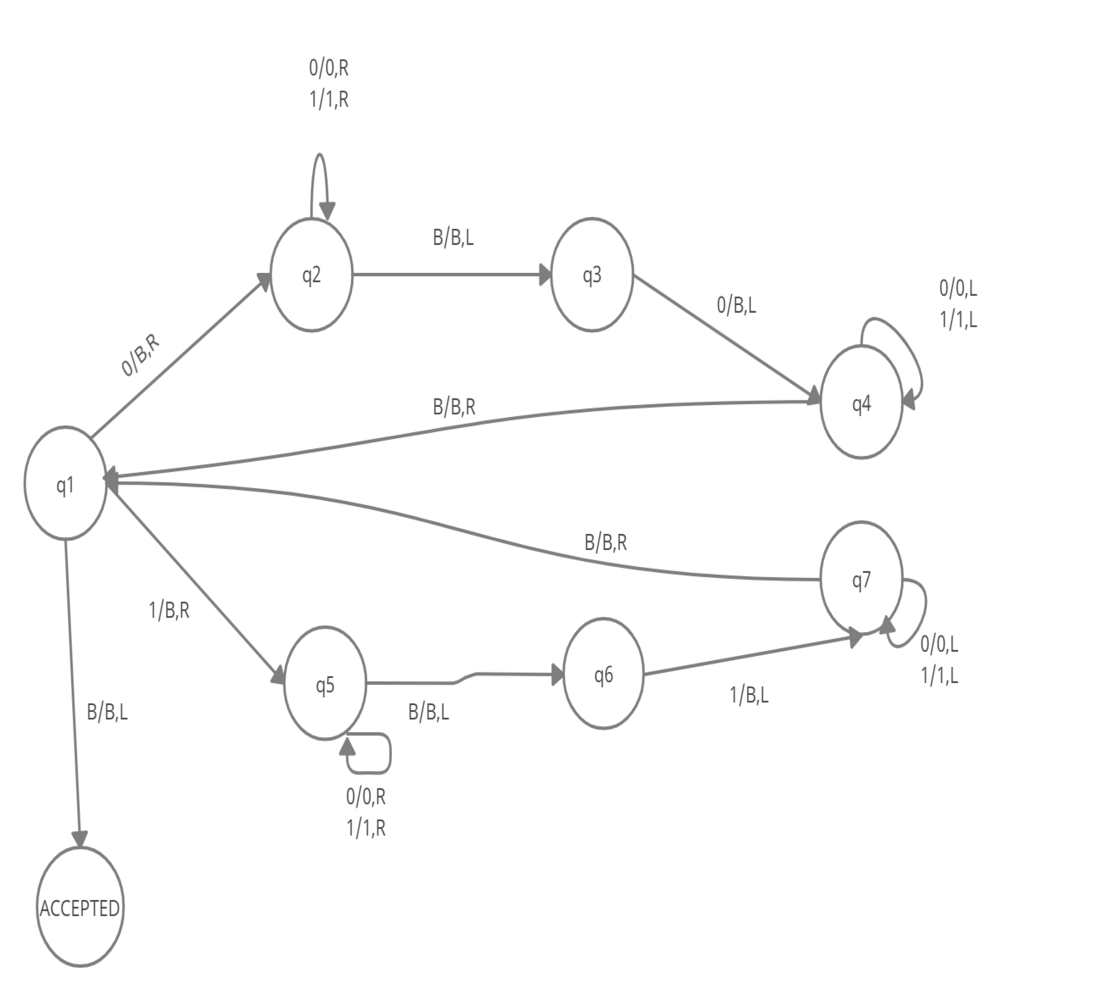Open in App
Not now

## Related Articles

• Write an Interview Experience

# Construct a Turing Machine for language L = {wwr | w ∈ {0, 1}}

• Difficulty Level : Expert
• Last Updated : 03 Jan, 2023

Prerequisite – Turing Machine

The language L = {wwr | w ∈ {0, 1}} represents a kind of language where you use only 2 character, i.e., 0 and 1. The first part of language can be any string of 0 and 1. The second part is the reverse of the first part. Combining both these parts out string will be formed. Any such string which falls in this category will be accepted by this language. The beginning and end of string is marked by \$ sign. For example, if first part w = 1 1 0 0 1 then second part wr = 1 0 0 1 1. It is clearly visible that wr is the reverse of w, so the string 1 1 0 0 1 1 0 0 1 1 is a part of given language.

Examples –

```Input : 0 0 1 1 1 1 0 0
Output : Accepted
Input : 1 0 1 0 0 1 0 1
Output : Accepted```

Basic Representation –Assumption: We will replace 0 by Y and 1 by X.

Approach Used – First check the first symbol, if it’s 0 then replace it by Y and by X if it’s 1. Then go to the end of string. So last symbol is same as first. We replace it also by X or Y depending on it. Now again come back to the position next to the symbol replace from the starting and repeat the same process as told above. One important thing to note is that since wr is reverse of w of both of them will have equal number of symbols. Every time replace a nth symbol from beginning of string, replace a corresponding nth symbol from the end.

• Step-1: If symbol is 0 replace it by Y and move right, Go to state Q2 If symbol is 1 replace it by X and move right, Go to state Q1
• Step-2: If symbol is 0 replace it by 0 and move right, remain on same state If symbol is 1 replace it by 1 and move right, remain on same state ——————————————————————- If symbol is X replace it by X and move right, Go to state Q3 If symbol is Y replace it by Y and move right, Go to state Q3 If symbol is \$ replace it by \$ and move right, Go to state Q3
• Step-3: If symbol is 1 replace it by X and move left, Go to state Q4 If symbol is 0 replace it by Y and move left, Go to state Q5
• Step-4: If symbol is 1 replace it by 1 and move left If symbol is 0 replace it by 0 and move left Remain on same state
• Step-5: If symbol is X replace it by X and move right If symbol is Y replace it by Y and move right Go to state Q0
• Step-6: If symbol is X replace it by X and move right If symbol is Y replace it by Y and move right Go to state Q6 ELSE Go to step 1
• Step-7: If symbol is X replace it by X and move right, Remain on same state If symbol is Y replace it by Y and move right, Remain on same state If symbol is \$ replace it by \$ and move left, STRING IS ACCEPTED, GO TO FINAL STATE Q7Another solution for the given problem:

If we look at the problem ,it is nothing but an even palindrome so the following is an another solution for the given language. Here I have consider Blank as B. The following turing machine checks that the language L = {wwr | w ∈ {0, 1}} is a palindrome or not.

• Step 1- if first symbol is “0” then it replace by the blank symbol goes to the right till it finds the blank and by checking that the symbol is blank or not .
• Step2- after getting the last symbol go to the one step left and check that symbol it is “0” or not and
• step3- if the last symbol is “0” then replace it with blank symbol and keep going left until it find the blank symbol
• step4- after finding the left most blank symbol go one step right and check it “0” or “1”
• Step 5- if we get “1” then replace it by the blank symbol and goes to the right until it find the blank symbol.
• Step 6- if it find the blank symbol then take one step left and check that symbol is “1” or not .If it is “1” then replace it with the blank symbol.
• step7- after replacing it goes to the left and find the blank symbol after finding the blank symbol take one step right and check if the symbol is “1” or “0”
• Step 8- repeat the above steps until the whole string become blank .My Personal Notes arrow_drop_up